# A tour of TSP heuristics## Preliminaries

### Modelisation

Let $G = (V, {E})$, where $V$ is a set of vertices and a set of arcs, be a undirected weighted complete graph:

• undirected: every ${e} \in {E}$ is a 2-set ${e} = \{u, v\}$, representing a two-way direction between $u \in V$ and $v \in V$.
• weighted: every ${e} = (u, v) \in {E}$ has a weight $d(u, v)$ (the distance from $u$ to $v$).
• complete: $G$ contains all possible arcs from a vertex to another.

Travelling Salesman Problem (TSP): find a cycle (or: tour) in $G$ visiting all vertices with minimum total weight

In this notebook we will restrict ourself to the Euclidean TSP, in which each vertex is a 2D point and $d$ is the euclidean distance between two points.

### Data structures

We will use a numpy array of size $n\times 2$ to store $n$ points. We will test our algorithms on random points:

In :
import numpy as np
from functools import cache

@cache # to compare algorithms on same data
def random_cities(n: int) -> np.array: # generate n points (x, y) with 0 <= x < 1000 and 0 <= y < 400
return np.random.uniform(0, (1000, 400), size=(n, 2))

random_cities(4)

Out:
array([[487.44409549, 223.65698794],
[351.34299086, 230.0642247 ],
[701.11985127, 277.64206405],
[415.22511322, 386.98932959]])
In :
CITIES = random_cities(40) # example used in this notebook


A cycle will be a list of points containing the vertices in the order they appear in the cycle. The length of a cycle is the sum of the euclidean distances between each vertex and the next:

In :
def d(p, q):
return np.sum((np.array(p) - np.array(q))**2, axis=-1)**.5

def length_cycle(cycle: list) -> float:
return d(cycle, np.roll(cycle, 1, axis=0)).sum()

length_cycle(random_cities(4))

Out:
973.9284414702624

An algorithm for TSP will be implemented as a function with a set of points as argument and returning a cycle:

In :
def algo(points: np.array) -> np.array:
return ... # cycle


### Vizualisation

In :
import matplotlib.pyplot as plt
import time

def plot_cycle(cycle):
cycle = list(cycle)
# print(cycle)
plt.figure(figsize=(20, 6))
X, Y = zip(*(cycle + [cycle]))
plt.plot(X, Y, 'bo-')
plt.scatter(X, Y, s=300) # starting point

def test_points(algo, points):
t = time.time()
cycle = list(algo(points))
plot_cycle(cycle)
plt.suptitle(f"Cycle length: {length_cycle(cycle):.2f}\nTime: {time.time() - t:.2f}s", fontsize=20)

def test(algo, n): # test algorithm on n random cities
test_points(algo, random_cities(n))

def plot_cycles(cycles, params):
n = len(cycles)
n_row = (n+1)//2
_, axes = plt.subplots(n_row, 2, figsize=(20, 5*n_row))
for i, cycle in enumerate(cycles):
cycle = list(cycle)
X, Y = zip(*(cycle + [cycle]))
a = axes.flat[i]
a.plot(X, Y, 'bo-')
a.scatter(X, Y, s=300) # starting point
a.set_title(f"Cycle length: {length_cycle(cycle):.2f} ({params[i]})", fontsize=20)


We will also take a look at a real graph, containing the 18 most populated cities in France:

In :
import folium # for map vizualization
import pandas as pd

def test_france(algo, n):
f = folium.Figure(width=800, height=400)
m = folium.Map(location=[46.3833, 4.91667], zoom_start=5)
df_big = df.sort_values(by=['ville_population_2012'])[["ville_nom", "ville_population_2012", "ville_latitude_deg", "ville_longitude_deg"]][-n:]
for i in range(len(df_big)):
# print(df.loc[i, "ville_latitude_deg"], df.loc[i, "ville_longitude_deg"])
city = df_big.iloc[i]
folium.CircleMarker(
location=[city["ville_latitude_deg"], city["ville_longitude_deg"]],
popup=city["ville_nom"] + " (" + str(city["ville_population_2012"]) + ")",
icon=folium.Icon(color="green"),
color="#3186cc",
fill=True,

points = df_big[["ville_latitude_deg", "ville_longitude_deg"]].to_numpy()
cycle = list(algo(points))
cycle.append(cycle)
return f


## Exact algorithms

### Brute force

The most simple solution is to consider all possible cycles and keep the minimum. This amounts to enumerate all permutations of the vertices:

In :
from itertools import permutations

def all_cycles(cities):
for perm in permutations(cities[1:]):
yield [cities] + list(perm) # first city is fixed

def algo_brute_force(cities):
cycles = all_cycles(cities)
return min(cycles, key=length_cycle)

test(algo_brute_force, 8)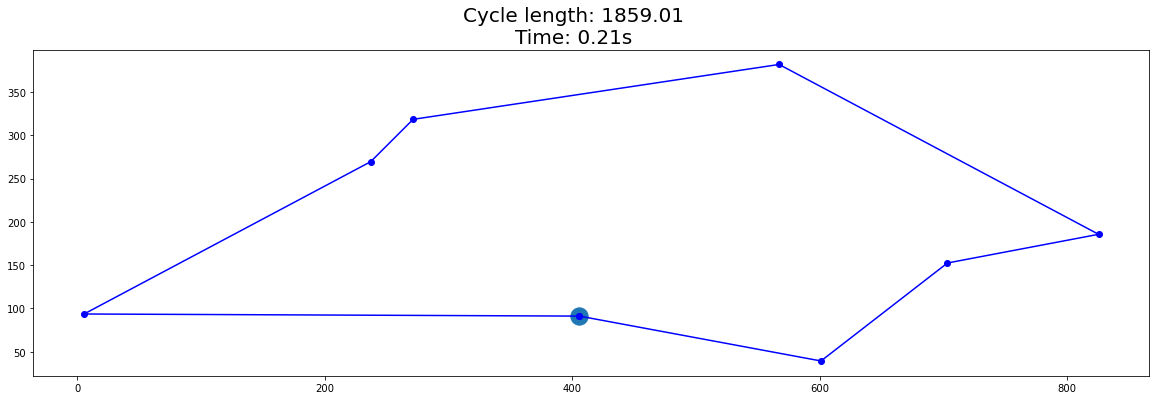The time complexity is O($n!$) (number of permutation of the $n$ cities), which is horrendous and unusable for $n > 10$.

In :
test_france(algo_brute_force, 8)

Out:

### Dynamic programming (Held–Karp algorithm)

Assume that $V = \{0, ..., n - 1\}$. Let $S \subseteq \{1, ..., n - 1\}$ and $v \notin S$.
Let $dp[S, v]$ be the length of a shortest cycle starting at $0$, visiting once every vertex of $S$, and ending in $v$.
We use the following induction: $$dp[\emptyset, v] = d(0, v)$$ $$dp[S, v] = \min_{u \in S} dp[S - u, u] + d(u, v)$$

In :
def algo_dp(points):
def dS(u, v):
return d(points[u], points[v])

@cache
def dp(S, v):
if len(S) == 0:
return dS(0, v)
return min(dp(S - {u}, u) + dS(u, v) for u in S)

def pred(S, v):
yield points[v]
if len(S) == 0:
return points
u = min((dp(S - {u}, u) + dS(u, v), u) for u in S)
yield from pred(S - {u}, u)

S = frozenset(range(len(points))) - {0}
return list(pred(S, 0))

test(algo_dp, 10)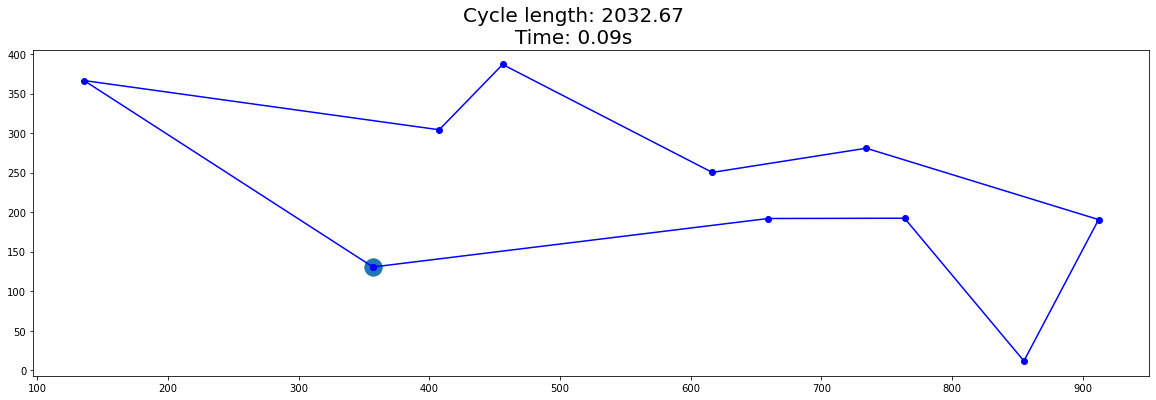In :
test_france(algo_dp, 15)

Out:

Unfortunately the complexity is still exponential: O($n2^n$) since we need to compute $dp[S, v]$ for $S \subseteq \{1, ... n - 1\}$ ($2^{n - 1}$ subsets) and $v \in V$ ($n$ vertices).

TODO

## Greedy algorithms

### Nearest neighbors

The nearest neighbors algorithm consists in starting in an arbitrary vertex then iteratively visiting the closest non-visited vertex.

In :
def closest(i, points):
p = points[i].copy()
points[i] = np.inf
j = np.argmin(np.sum((points - p)**2, axis=1))
return j, p

def algo_nn(points):
import copy
j = 0
points_copy = copy.deepcopy(points)
for _ in range(len(points)):
j, p = closest(j, points_copy)
yield p
yield points

test(algo_nn, 40)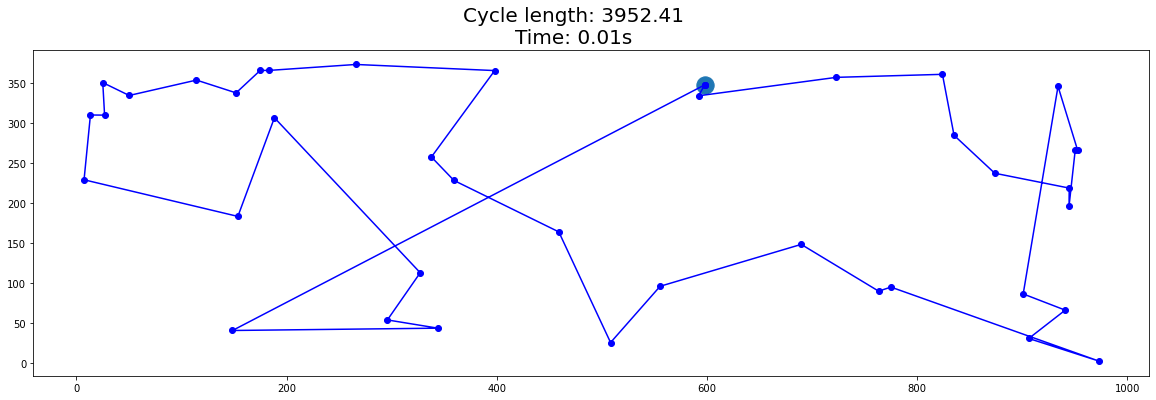### Local search: uncrossing for nearest neighbors (2-opt)

We can observe that there are often "big jumps" in the nearest neighbors solution, like this:

In :
points = np.array([[0, 0], [300, 0], [100, 300], [800, 300]], dtype=float)
test_points(algo_nn, points)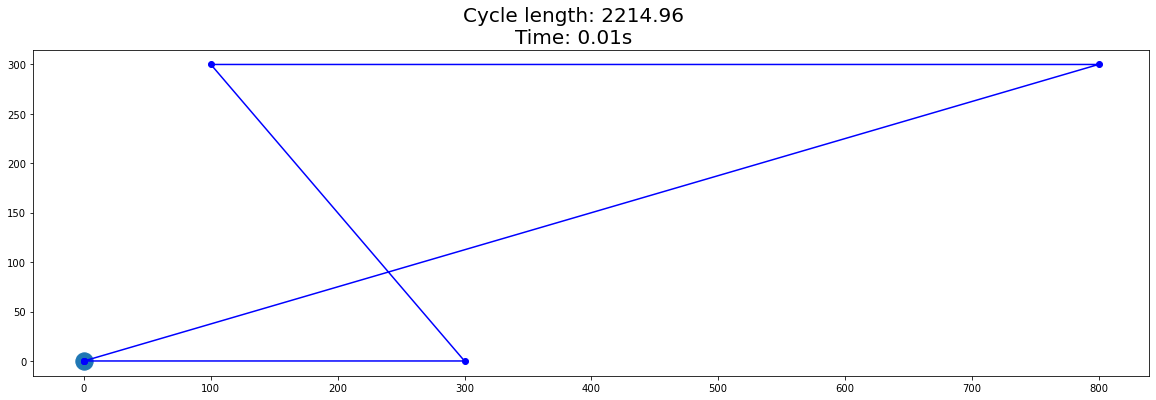This can be improved by "uncrossing":

In :
def uncrossing(p): # uncross every pair of edges in path p
n = len(p)
b = False # has an improvment been made?
for i in range(n):
for j in range(i + 1, n):
if d(p[i], p[(i+1)%n]) + d(p[j], p[(j+1)%n]) > d(p[i], p[j]) + d(p[(i+1)%n], p[(j+1)%n]):
p[i + 1:j+1] = p[i + 1:j+1][::-1]
b = True
return b

def algo_uncrossing(algo):
def aux(points):
path = list(algo(points))
while uncrossing(path):
pass
return path
return aux

test_points(algo_uncrossing(algo_nn), points)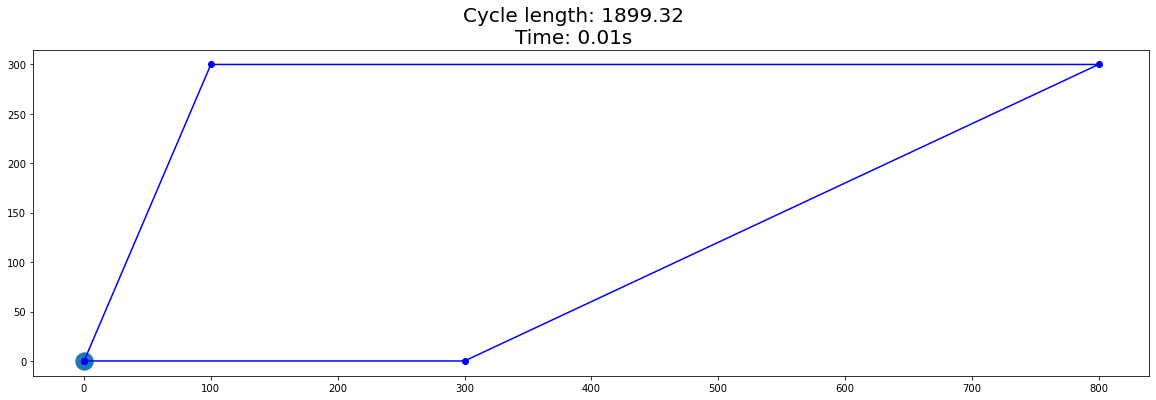The loop while uncrossing(path) is terminating since the length of path is decreasing by at least $c$ for each iteration, where $c$ is the minimum of non-zero distance gained by uncrossing any two edges.

In :
cycles = [algo_nn(CITIES), algo_uncrossing(algo_nn)(CITIES)]
plot_cycles(cycles, ["NN", "NN uncrossing"])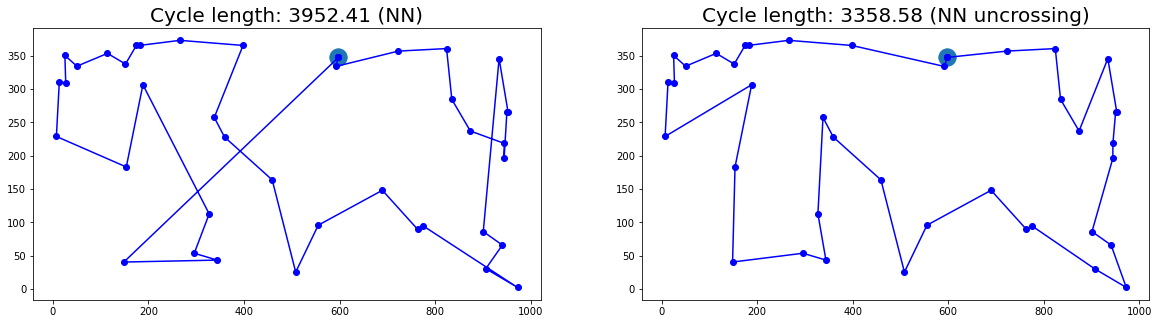## Genetic algorithm

We will apply the following genetic algorithm:

1. Generate an initial population: a set of cycles randomly generated
2. Keep only the best individuals: the shortest half
3. Crossover: combine two cycles into one
4. Mutate the individuals: exchange two vertices at random from each cycle, with some probability mutation_rate
In :
def initial_population(points, sz_pop):
return [list(map(tuple, np.random.permutation(points))) for _ in range(sz_pop)]

def crossover(cycle1, cycle2):
n = len(cycle1)
cycle = cycle1[:n//2]
for p in cycle2:
if p not in cycle:
cycle.append(p)
return cycle

def mutate(pop, mutation_rate):
for i in range(len(pop)):
if np.random.random() < mutation_rate:
j, k = np.random.choice(range(len(pop[i])), 2)
pop[i][j], pop[i][k] = pop[i][k], pop[i][j]

def algo_genetic(points, sz_pop=300, n_iterations=300, mutation_rate=0.5):
Y = [] # length of the best cycle for each iteration
pop = initial_population(points, sz_pop)
for _ in range(n_iterations):
pop.sort(key=length_cycle)
Y.append(length_cycle(pop))
pop = pop[:len(pop)//2]
pop.extend([crossover(pop[i-1], pop[i]) for i in range(len(pop))])
mutate(pop, mutation_rate)
return min(pop, key=length_cycle), (Y, mutation_rate)

points = random_cities(40)
proba_mut = [0.2, 0.4, 0.5, 0.6, 0.7, 0.9]
from multiprocessing import Pool
with Pool(6) as pool:
def f(p):
return algo_genetic(points, mutation_rate=p)
cycles, Y_list = zip(*pool.map(f, proba_mut))
plt.figure()
for Y, mut in Y_list:
plt.plot(range(len(Y)), Y, label=mut)
plt.legend()
plot_cycles(cycles, proba_mut)
plt.show()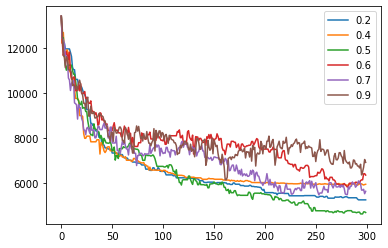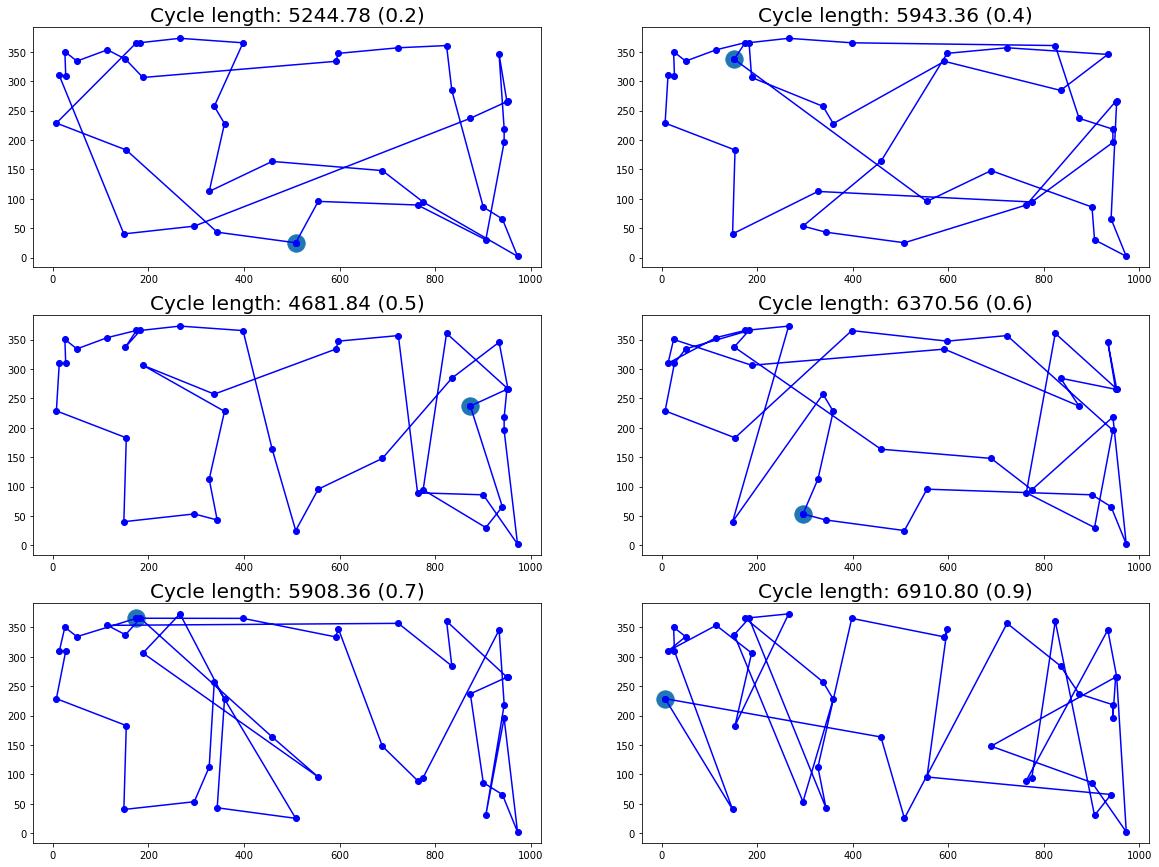In :
cycle = algo_genetic(CITIES, sz_pop=1000, n_iterations=1000)
cycle_uncross = cycle[:]
uncrossing(cycle_uncross)
plot_cycles([cycle, cycle_uncross], ["Genetic", "Genetic uncrossing"])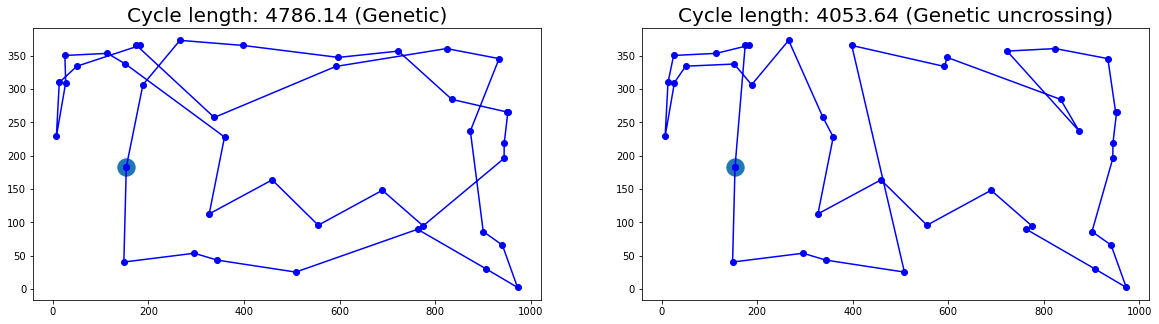## Summary

Algorithm Cycle length for 40 cities
Genetic + uncrossing 4053
Nearest neighbor + uncrossing 3358
Branch & bound
In [ ]:
# plt.rcParams["figure.figsize"] = (20,3)
# n = 40
# points = random_cities(n)
# for algo, name in [(algo_genetic, "genetic"), (algo_uncrossing(algo_nn), "nn uncrossing")]:
#     print(name)
#     print(length_cycle(algo(points)))